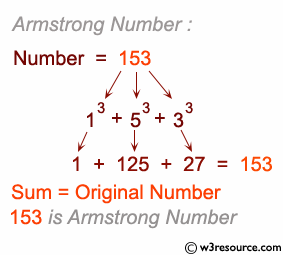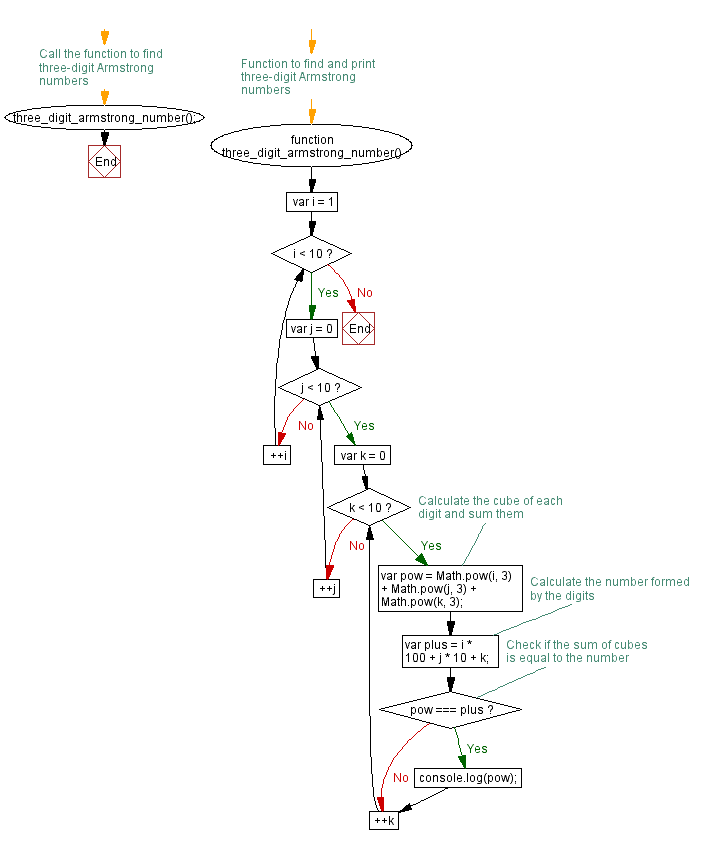# JavaScript: Find the armstrong numbers of 3 digits

## JavaScript Conditional Statement and loops: Exercise-9 with Solution

Write a JavaScript program to find the armstrong numbers of 3 digits.

Note : An Armstrong number of three digits is an integer such that the sum of the cubes of its digits is equal to the number itself. For example, 371 is an Armstrong number since 3**3 + 7**3 + 1**3 = 371.

Pictorial Presentation:Sample Solution:-

HTML Code:

``````<!DOCTYPE html>
<html>
<meta charset=utf-8 />
<title>Three digit armstrong numbers</title>
<body>

</body>
</html>
```
```

JavaScript Code:

``````function three_digit_armstrong_number()
{
for (var i = 1; i < 10; ++i)
{
for (var j = 0; j < 10; ++j)
{
for (var k = 0; k < 10; ++k)
{
var pow = (Math.pow(i,3) + Math.pow(j,3) + Math.pow(k,3));
var plus = (i * 100 + j * 10 +  k);
if (pow == plus)
{
console.log(pow);
}
}
}
}
}
three_digit_armstrong_number();
```
```

Sample Output:

```153
370
371
407
```

Flowchart:Live Demo:

See the Pen javascript-conditional-statements-and-loops-exercise-9 by w3resource (@w3resource) on CodePen.

Improve this sample solution and post your code through Disqus

What is the difficulty level of this exercise?

Test your Programming skills with w3resource's quiz.

﻿

## JavaScript: Tips of the Day

Creates an array of elements, ungrouping the elements in an array produced by zip and applying the provided function

Example:

```const tips_unzip = (arr, fn) =>
arr
.reduce(
(acc, val) => (val.forEach((v, i) => acc[i].push(v)), acc),
Array.from({
length: Math.max(...arr.map(x => x.length))
}).map(x => [])
)
.map(val => fn(...val));

console.log(tips_unzip([[2, 15, 200], [3, 25, 300]], (...args) => args.reduce((acc, v) => acc + v, 0)));
```

Output:

```[5, 40, 500]
```